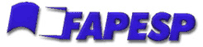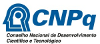## Program

 Mon 28th Jul Tue 29th Jul Wed 30th Jul Thu 31th Jul Fri 1st Aug 8:00-9:00 registration 9:00-9:40 W. Oliva M. Abreu M. Alves K. de Resende P. Henriques 9:50-10:30 D. Gomes S. Sandon M. Batoréo F. Dickstein G. Siciliano 10:40-11:20 C. Rocha U. Hryniewicz J. Boldrini M. Teixeira P. Cordaro 11:20-14:00 14:00-14:40 P. Girão J. Weber D. Lopes J. Vetois 14:50-15:30 J. Matias M. Jardim J. Silva A. de Carvalho 15:30-16:00 coffee coffee coffee coffee 16:00-16:40 D. Martinez L. Macarini F. Costa 19:30- Dinner

## Dinner - Tuesday 29th July

The dinner will be held at Bovinus Churrascaria, a barbecue restaurant, for R\$ 90,00 each. Drinks, taxes and transport included.

## Transport from/to hotel

There will be mini-buses from hotel Howard Johnson Faria Lima Inn to the conference. On Monday, due to the registration, the bus leaves the hotel earlier at 7:30am. The other days it leaves at 8:30am. After the last talk of each day, the mini-buses can take the participants back to the hotel.

## Titles and Abstracts

• Carlos Rocha (IST)

Unstable manifold decompositions of Sturm global attractors.

We study global attractors for dissipative semiflows generated by scalar reaction-diffusion equations defined on an interval with Neumann boundary conditions. We assume that all equilibrium solutions are hyperbolic. Due to a gradient-like structure the global attractor then possesses a dynamical decomposition into finitely many disjoint invariant sets, the unstable manifolds of the equilibria. We explore this decomposition to describe the global attractor as a finite regular CW-complex. This is based on a joint work with B. Fiedler.

• Miguel Abreu (IST)

Toric constructions of monotone Lagrangian submanifolds in CP^2 and CP^1 x CP^1

Motivated by a toric model of the Chekanov-Schlenk exotic Lagrangian torus in CP^2, I will present a toric construction of certain Lagrangian submanifolds and apply it to obtain some old and new monotone examples in CP^2 and CP^1 x CP^1. This is joint work with Agnès Gadbled.

• Diogo Gomes (IST)

Introduction to the regularity theory for mean-field games

In this talk we will cover various aspects of the regularity theory for mean-field games. We will start with a brief introduction to Hamilton-Jacobi equations and viscosity solutions. Then we present several techniques to obtain regularity for Hamilton-Jacobi equations and Fokker-Planck equations. In addition to maximum principle and energy type estimates, we will introduce more recent tools such as the non-linear adjoint method. Then we derive a number of a-priori estimates for stationary and time-dependent mean-field games. Finally, gathering the previous results we establish existence of smooth solutions for various classes of mean-field games.

• David Martinez (IST)

Compact foliations in Poisson geometry

A Poisson manifold is foliated by symplectic leaves; the behavior of the (possibly singular) foliation can be rather complicated. We introduce `compact type conditions' for Poisson manifolds so that their leaf spaces become orbifolds (i.e, our conditions can be thought of as analogs of finite holonomy for compact foliatons). We will also see how these orbifolds are endowed with integral affine structures. This is joint work with M. Crainic and R. L. Fernandes.

• José Matias (IST)

Some results in the Calculus of Variations for integrands with linear growth in the context of A-quasiconvexity

I will present an overview of ongoing work in some problems in the Calculus of Variations, namely lower-semicontinuity and relaxation problems, differential inclusions, characterization of generalized Young-measures and homogenization problems, that have in common the linear growth of integrands and the context of A-quasiconvexity.

• Pedro Henriques (IST)

The General Case of the Inverse Problem of Variational Calculus with Non-holonomic Constraints

We will analyse the general case of the inverse problem of variational calculus with nonholonomic constraints. Our analysis focuses on variational problems that seek to optimize linear functionals given by n-form actions with Lagrangians with several independent and dependent variables.

• Pedro Girão (IST)

On the global uniqueness for the Einstein-Maxwell-scalar field system with a cosmological constant

Given spherically symmetric characteristic initial data for the Einstein-Maxwell-scalar field system with a cosmological constant \$\Lambda\$, with the data on the outgoing initial null hypersurface given by a subextremal Reissner-Nordström black hole event horizon, we study the future extendibility of the corresponding maximal globally hyperbolic development as a Lorentzian manifold. This is joint work with João Costa, José Natário and Jorge Silva.

• Jorge D. Silva (IST)

Nonlinear Schrödinger equation with time dependent potential: large time properties

We present recent results on large time behaviour of solutions to the nonlinear Schrödinger equation with time dependent external potential and defocusing nonlinearity. The potential is assumed to grow at most quadratically in space, uniformly for all time, for which a typical example is a (possibly anisotropic) harmonic potential with bounded coefficients in time. We start by presenting a global in time well posedness result without further assumptions on the potential, with a general exponential growth control of its first order derivatives and momenta. As a consequence, these yield a double exponential growth rate of the higher Sobolev norms and momenta. We also show that if the potential is harmonic and isotropic, with coefficients decaying sufficiently fast in time, then there is scattering, the Sobolev norms remain bounded and momenta grow polinomially in time.This is joint work with Rémi Carles.

• Waldyr M. Oliva (IST/IME-USP)

Um pouco sobre a vida matemática de Orlando Francisco Lopes

Trata-se de resumir a colaboração do Orlando do ponto de vista matemático, tanto na pesquisa como no ensino, pelos departamentos brasileiros e estrangeiros por onde passou nos seus fecundos anos de atividade acadêmica.

• Paulo D. Cordaro (IME-USP)

Strong unique continuation for systems of complex vector fields

In this work we study the property of strong unique continuation, at a given point, for Gevrey solutions to homogeneous systems of PDE defined by complex, real-analytic vector fields in involution. We show that when the system is minimal at the point then the strong unique continuation property holds for Gevrey solutions of order between one and two and, furthermore, when the minimality property fails to hold then there are non trivial Gevrey flat solutions of any given order bigger than one. The case of Gevrey order bigger than two is also studied for some particular classes of involutive systems. Authors: R. Barostichi, P.D. Cordaro and G. Petronilho.

• Gaetano Siciliano (IME-USP)

Existence results for an elliptic equation involving the fractional Laplacian

Abstract

• Marco Antônio Teixeira (UNICAMP)

On nonsmooth dynamical systems and singular perturbations problems

This talk proposes to discuss the connection between the regularization process of non-smooth vector fields (Filippov Systems) and the singular perturbation problems. The objective is to explore the local behavior of systems around typical singularities.

• Marcos B. Jardim (UNICAMP)

Instantons and their moduli spaces

I will present some basic fact about instantons on 4-dimensional Riemannian manifolds and their moduli spaces. I will then focus on the case of instantons over the 4-sphere, and explain their relation with complex geometry via twistor theory. I will conclude with recent results on the moduli spaces of instanton bundles on CP^3.

• Joachim Weber (UNICAMP)

Morse Homology for semi-flows and the backward Lambda Lemma

Consider a closed Riemannian manifold M and the associated heat flow on the loop space of M. We show how that Morse homology of the heat flow, as constructed in arXiv:1003.4350, naturally represents singular homology of the free loop space. The key step is to construct a Morse filtration of the loop space. Here we use Conley's theory of index pairs -- in our case associated to isolated fixed points of the heat flow, that is closed geodesics in M. Our attempts to calculate the Conley homotopy index resulted in the discovery arXiv:1210.3897 of a *backward* lambda lemma for the heat flow. (Recall that in fact the heat flow is only a forward semi-flow; how much backward time information it carries nevertheless, seems rather unstudied and unknown.) To summarize, these constructions generalize Morse homology theory from the well known case of flows on Banach manifolds to semi-flows. In particular, they establish a natural isomorphism to singular homology. This construction of the isomorphism is new, even for flows; see arXiv:1211.2180 for an outline.

• José Luiz Boldrini (UNICAMP)

Stationary solutions of a singular Navier-Stokes-enthalpy-heat conduction system

We present a result on existence of stationary solutions of a system coupling a set of singular Navier-Stokes equations to a enthalpy-heat condition equation. This may model the solidification process of certain classes of materials by taking in consideration the possibility of flow in the melt; thus, the singular Navier-Stokes equations only holds in the a priori unknown molten region and one has a free-boundary value problem to be solved. To obtain solutions for such system, we initially consider a sequence of approximate problems associated to an appropriate regularizations of the original one, which are obtained by a suitable modification in order that the approximate equations for the flow hold in the entire domain. After analyzing these approximate problems, by using compactness arguments, we take limits and obtain generalized solutions of the original problem. These results were obtained by joint work with S.A. Lorca P. (Universidad de Tarapaca, Chile) and H. Soto (Universidad de La Frontera, Chile).

• Ketty A. de Resende (UNICAMP)

Spectral sequences and the underlying dynamical information they carry

In this talk we present the topological tools found in Conley's index theory, in order to explore different filtered chain complexes generated by critical points and compute their spectral sequence using the sweeping algorithm. We then show that this process corresponds to continuation properties of the associated flow.

• Flávio Dickstein (UFRJ)

Standing waves of the complex Ginzburg-Landau equation

Abstract

• Umberto Hryniewicz (UFRJ)

A Poincaré-Birkhoff theorem for tight Reeb flows on the three sphere

In 2005 Angenent proved that a simple closed geodesic with inverse Poincaré rotation number different from one implies the existence of infinitely many closed geodesics on a Riemannian 2-sphere. This is an example of a Poincaré-Birkhoff type phenomenon, not for area-preserving maps but for three dimensional Reeb flows. In this talk I will explain the results of joint work with Pedro A. S. Salomão and Al Momin, which use the machinery of contact homology to prove such a theorem for Reeb flows on 3-sphere. In particular, we generalize Angenent's result for non-reversible Finsler metrics carrying a pair of special closed geodesics. We will also discuss how these of results can be combined with the analysis of Nancy Hingston to provide a new proof of a famous result of Bangert-Franks stating that every Riemannian metric on the 2-sphere carries infinitely many closed geodesics.

• Leonardo Macarini (UFRJ)

Infinitely many periodic orbits for exact magnetic flows on surfaces

We consider exact magnetic flows on a closed surface and prove that for almost every energy level k below Mane's critical value of the universal covering there are infinitely many periodic orbits with energy k. This is joint work with A. Abbondandolo, M. Mazzucchelli and G. Paternain.

• Sheila Sandon (CNRS)

On bi-invariant metrics on the contactomorphism group

While in symplectic topology a famous bi-invariant metric on the group of Hamiltonian diffeomorphisms (the Hofer metric) and a few variant on it have been known for more than 20 years, it has been only quite recently that the first bi-invariant metrics have been discovered on the group of contact diffeomorphisms. The first such metric was constructed by me in my thesis (2009) for the contact manifold R^2n x S^1, and this construction was then extended in 2012 by Zapolsky and Fraser-Polterovich-Rosen to other contact manifolds with periodic Reeb flow. In the same year in collaboration with Vincent Colin we defined a bi-invariant metric (the oscillation metric) on the universal cover of the contactomorphism of any contact manifold. In my talk I will review these constructions and discuss their relations with other rigidity phenomena in contact topology such as the notions of orderability (introduced by Eliashberg and Polterovich in 2000) and contact squeezing (as first studied by Eliashberg-Kim and Polterovich in 2006).

• Fernando P. Costa (Univ. Aberta - Porto)

Mathematical aspects of ``cluster eating'' equations

Abstract

• Alexandre N. de Carvalho (ICMC-USP)

Dynamical systems and their attractors under perturbations

In this lecture we recall the definitions of autonomous and non autonomous Dynamical Systems as well as their different concepts of attractors. After that we introduce the different notions of robustness of attractors under perturbation (Upper semicontinuity, Lower semicontinuity, Topological structural stability and Structural stability) and give conditions on the dynamical systems so that robustness is attained.

• Marta Batoréo (IMPA)

On non-contratible periodic orbits of symplectomorphisms. We present a result on the existence of infinitely many non-contratible periodic orbits of certain symplectomorphisms isotopic to the identity if they admit at least one hyperbolic periodic orbit. It holds for a certain class of closed symplectic manifolds and the main tool used in the proof is Floer-Novikov homology for non-contractible periodic orbits. The proof uses a key feature of hyperbolic periodic orbits.

• Jerome Vetois (University of Nice - Sophia Antipolis)

Sign-changing blow-up for scalar curvature type equations

I will present a joint work with Frederic Robert (University of Lorraine) on the existence of blowing-up solutions which change sign for elliptic equations of scalar curvature type on a compact Riemannian manifold. Our results complete previous results showing the existence or non-existence of blowing-up solutions for this type of equation. In particular, I will show how the picture for sign-changing blow-up differs from the case of positive solutions.

• Marcelo Alves (Université Libre de Bruxelles)

Contact homologies and topological entropy

In this talk I will present certain results that show how Contact homology, an invariant of contact manifolds which comes from Symplectic Field Theory, can be used to obtain estimates for the topological entropy of Reeb vector fields. The first connection between symplectic invariants and topological entropy appears in the work of Macarini and Schlenk, who used Lagrangian Floer homology to obtain positivity of topological entropy for smooth Reeb flows in the unit tangent bundle of a manifold with "complicated" topology. Building on their work, we substitute their use of Lagrangian Floer homology by Cylindrical contact homology and Legendrian contact homology; these have the advantage of being defined for contact manifolds where the Lagrangian Floer homology cannot be defined, and to allow estimates for topological entropy in the case of finite differentiability. As an application, we obtain a plethora of new examples of contact 3-manifolds for which every Reeb flow has positive topological entropy.

• Débora Lopes (UFSE)

Pontos parcialmente umbílicos em famílias a um parâmetro de hipersuperfícies imersas em \$\R^4\$

Abstract

## Realization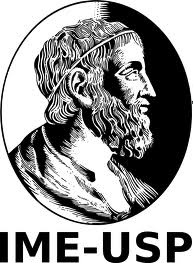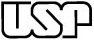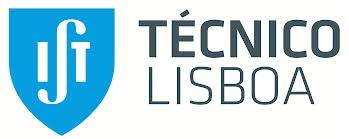## Supported by